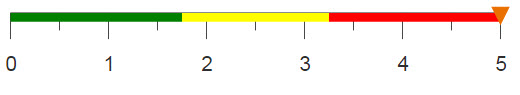# Extracting loadings/eigenvectors from your PCA

Using 1010data's g_function, g_pca(G;S;XX;Z), you can create a model that corresponds to the principal component analysis of one or more variables. However, in order to determine the loadings/eigenvectors you need to use param(M;'evecs';J I).

## Difficulty## Objective

You have completed a principal component analysis using g_pca(G;S;XX;Z). Now you are interested in determining the loadings, otherwise known as eigenvectors. Using the param function with the value 'evecs' as the argument for the P parameter, you can calculate the individual elements of each eigenvector, but you want to create a matrix containing all of the loadings.

## Solution

```<block name="eigenvector_table"
model_vars="age,duration,previous,empvarrate,hsng,h_unk,def">
<base table="pub.demo.mleg.uci.bankmarketing"/>
<willbe name="yy" value="y='yes'"/>
<willbe name="hsng" value="housing='yes'"/>
<willbe name="h_unk" value="housing='unknown'"/>
<willbe name="def" value="default='yes'"/>
<willbe name="model_pca" value="g_pca(;;{@model_vars};'method''corr')"/>
<sel value="i_<=csl_len('{@model_vars}')"/>
<willbe name="row" value="i_()"/>
<for i="1" to="{csl_len('{@model_vars}')}">
<willbe name="evec_{@i}" value="param(model_pca;'evecs';{@i} row)"/>
</for>
<colord cols="evec_*"/>
</block>```

## Discussion

The first part of this solution is discussed in the Principal Component Analysis tutorial, which can be accessed from the Further reading links below. Once you have your model, you can use the param function to determine the weights of the components. However, with this function you need to calculate each element individually, specifying the column and element for each eigenvector.

Storing your model variables or column names in a block variable allows you to systematically calculate all loadings. You can count the number of elements in your block variable, model_vars, using csl_len(X), and therefore determine the number of variables included in your model. There will only be as many eigenvectors and elements within as there are variables in your model, therefore, a selection is performed to limit the number of rows to this number. You then create a column that denotes the row number.

Using a <for> loop, you can create a column for each eigenvector. param(M;'evecs';J I) takes two numbers in the last argument. The first is J, for the Jth eigenvector, and I, for the Ith element in the Jth eigenvector. Therefore, you can use the current iteration number, {@i}, for J and the current row number, row, for I.

After a <colord> statement, you are left with the matrix containing the loadings/eigenvectors for the model produced by g_pca(G;S;XX;Z).
Note: Notice that you can use evec_* instead of writing out each of the column names and it will include any column name that starts with "evec_".

## Further reading

If you would like to learn more about the functions and operations discussed in this recipe, click on the links below:

param(M;P;I)

g_pca(G;S;XX;Z)

Principal Component Analysis